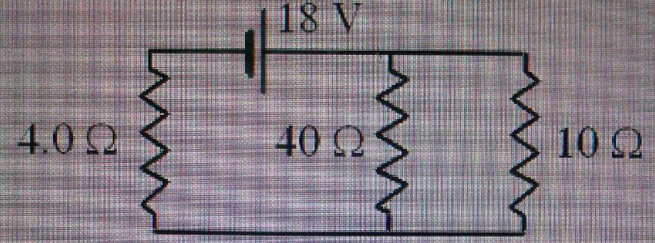# Problem: Determine the power dissipated by the 40 ohm resistor in the circuit shown. (the values from left to right at 4.0 ohm, 40 ohm, 10 ohm, 18V)Possible answers are1. 9.0 W2. 27 W3. 4.5 W4. 3.6 W5. 14WPlease show your work.

###### FREE Expert Solution

Equivalent resistance for 2 resistors in parallel:

$\overline{){{\mathbf{R}}}_{{\mathbf{eq}}}{\mathbf{=}}\frac{{\mathbf{R}}_{\mathbf{1}}{\mathbf{R}}_{\mathbf{2}}}{{\mathbf{R}}_{\mathbf{1}}\mathbf{+}{\mathbf{R}}_{\mathbf{2}}}}$

Equivalent resistance for resistors in series:

$\overline{){{\mathbf{R}}}_{{\mathbf{eq}}}{\mathbf{=}}{{\mathbf{R}}}_{{\mathbf{1}}}{\mathbf{+}}{{\mathbf{R}}}_{{\mathbf{2}}}{\mathbf{+}}{\mathbf{.}}{\mathbf{.}}{\mathbf{.}}{\mathbf{+}}{{\mathbf{R}}}_{{\mathbf{n}}}}$

Ohm's law:

$\overline{){\mathbit{i}}{\mathbf{=}}\frac{\mathbf{V}}{\mathbf{R}}}$

Power:

$\overline{){\mathbf{P}}{\mathbf{=}}\frac{{\mathbf{V}}^{\mathbf{2}}}{\mathbf{R}}}$

The 40Ω and 10Ω resistors are in parallel.

They are all in series with the 4.0Ω resistor.

Req = 4.0 + (40)(10)/(40 + 10) = 12Ω

From Ohm's law, current, i = V/R

81% (239 ratings)###### Problem Details

Determine the power dissipated by the 40 ohm resistor in the circuit shown. (the values from left to right at 4.0 ohm, 40 ohm, 10 ohm, 18V)1. 9.0 W

2. 27 W

3. 4.5 W

4. 3.6 W

5. 14W